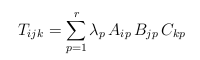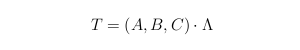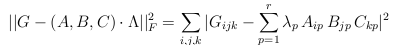# Tensor Package

## Introduction

We consider the decomposition of a tensor in a sum of rank-1 terms. This decomposition is generally referred to as "Canonical Polyadic" decomposition, and sometimes as "Parafac". In order to fix this discrepancy, many authors have adopted the "CP" acronym, which stands both for "Candecomp-Parafac" and for "Canonical Polyadic". See the introductory papers below for further details:

 P. Comon, ``Tensors: a brief introduction,''   IEEE Sig. Proc. Magazine, 31(3):44-53, May 2014. Preprint: hal-00923279

 P. Comon, X. Luciani, and A. L. F. de Almeida, ``Tensor Decompositions, Alternating Least Squares and other Tales,''  Jour. Chemometrics, 23:393--405, August 2009. Preprint: hal-00410057 or local preprint

In order to fix the ideas, let's take the case of 3rd order tensors. Once the three bases are chosen in each of the three vector spaces, the tensor is defined by a 3-way array. Its CP decomposition takes the form:where one can impose the columns of matrices A, B, and C to have unit norm columns. In compact notation, this is noted asThe minimal number of such rank-1 terms that are necessary to reconstruct tensor T exactly is called the rank of T. Suppose we are given the array T, and we wish to compute the CP decomposition. If the rank is smaller than the so-called expected dimension , then the CP is generally unique. However, noise may be present in measurements, so that the array may contain undesired contributions, which we may prefer to remove. In addition, noise may increase the rank beyond the latter bound, so that uniqueness may not be ensured. Hence, before computing the CP, it is often useful to reduce the rank.
• Rank reduction. Removing noise is standard in many applications, and can be performed by truncating the multilinear rank. This rank is a triplet of numbers, which correspond to the matrix rank of three possible flattening matrices (obtained by storing the 3rd order array values in matrix form); see  for complementary explanations and bibliographical references. The reduction of the multilinear rank implies (indirectly) tensor rank reduction. Denote G the new tensor obtained from T by multilinear rank reduction.
• Optimization. The next step consists of minimizing, i.e. a norm of the difference between actual tensor and model, for increasing values of r until the resulting difference is null. If the Frobenius norm is chosen, we minimize:The difficulty in minimizing this objective function lies in the fact  it is multimodal (there are local minima) and that there are many variables. Several algorithms have been developed to minimize this criterion:
• Alternating Least Squares (ALS). It is the least attractive as far as performances are concerned. But it is the easiest to program.
• Gradient descent. It can be implemented with fixed or variable step size. In practice, computing the locally optimal step size is unuseful and unnecessarily costly.
• Quasi-Newton. The BFGS implementation is quite efficient.
• Levenberg-Marquardt. The implementation presented in  performs quite well.
• Conjugate gradient. This is the implementation of Fletcher-Reeves, with regular reinitializations.
Each of these (standard) algorithms defines a direction of search, and a stepsize. In order to permit the algorithm to escape from local minima at a reasonable computational price, the Enhanced Line Seach (ELS) function has been developed; it computes the global minimum of the objective in any given direction. Because the objective function is polynomial, efficient routines are available and computationally little demanding. The largest part of the load consists of computing the polynomial coefficients. All this is done by the els.m function. For further details on the ELS algorithm, see:

 M. Rajih, P. Comon, R. Harshman, ``Enhanced Line Search: A Novel Method to Accelerate Parafac,'' SIAM Journal on Matrix Analysis Appl., 30(3):1148--1171, September 2008. Preprint: hal-00327595 or local preprint## General-purpose codes

Most of the following codes have been written or rewritten by X.Luciani during his post-doc with P.Comon at I3S in 2009:
• als.m   (Alternating  Least Squares)
• bfgs.m   (Quasi Newton)
• lm.m   (Levenberg-Marquardt)
• els.m    (Enhanced Line Search, for any of the previous iterations)
• main.m  (a main code, showing examples of how to call the previous functions)

References:

 P. Comon, X. Luciani, and A. L. F. de Almeida, ``Tensor Decompositions, Alternating Least Squares and other Tales,''  Jour. Chemometrics, 23:393--405, August 2009. Preprint: hal-00410057
 M. Rajih, P. Comon, R. Harshman, ``Enhanced Line Search: A Novel Method to Accelerate Parafac,'' SIAM Journal on Matrix Analysis Appl., 30(3):1148--1171, September 2008. Preprint: hal-00327595

## Codes for rank-1 deflation

• These codes have been developed by Alex P. da Silva under supervision of P.Comon:
• SeROAP
• THOSVD
• CE
• DCPD

References:

•  A. P. da Silva, P. Comon and A. L. F. de Almeida, ``An Iterative Deflation Algorithm for Exact CP Tensor Decomposition'', ICASSP, April 19-24, 2015, Brisbane, Australia.  hal-01071402 DOI: 10.1109/ICASSP.2015.7178714
• A. P. da Silva, P. Comon and A. L. F. de Almeida, ``Rank-1 Tensor Approximation Methods and Application to Deflation'', arxiv:1508.05273, August 21, 2015.
Shorter version published in:
 A. Pereira da Silva, P. Comon, and A. Lima Ferrer de Almeida, ``A finite algorithm to compute rank-1 tensor approximations,'' Sig. Proc. Letters, 23(7):959-963, July 2016. DOI: 10.1109/LSP.2016.2570862

• ## Codes for real nonnegative tensors

• Other codes for tensors with nonnegative entries, and decomposed into nonnegative rank-1 terms. These codes have been developed by J-P.Royer under supervision of N.Thirion and P.Comon, and include:
• bfgsP.m  (BFGS implementation of quasi-Newton)
Download the codes dedicated to tensors with nonNegative entries in a single zip archive.

Reference:
 J.P. Royer, N. Thirion and P. Comon, ``Computing the polyadic decomposition of nonnegative third order tensors'',  Signal Processing, Elsevier, vol.91, no.9, pp. 2159-2171, Sept. 2011. Preprint:  hal-00618729.

• When tensors have a large size, we developed special codes able to compress the data (low rank) while at the same time maintain nonnegativity. These codes have been developed by J.E.Cohen and R.Cabral-Farias under the supervision of P.Comon:

Download the codes dedicated to big nonnegative tensors in a single zip archive

Reference:
 J. E. Cohen, R. Cabral-Farias and P. Comon, ``Fast Decomposition of Large Nonnegative Tensors'',  IEEE Sig. Proc. Letters, vol.22, no.7, pp.862-866, July 2015. Preprint:  hal-01069069.

## Codes for coupled decompositions

• When several data sets are available in the form of tensors of different sizes with latent vectors related to each other through (possibly noisy) linear relations, joint flexible CP decomposition can be performed. In the contribution below, A Bayesian (MAP) approch is developed :

Reference:
 R. Cabral Farias, J. E. Cohen and P. Comon , ``Exploring multimodal data fusion through joint decompositions with flexible couplings'',  IEEE Trans. Signal Processing, vol.64, no.18, pp.4830-4844, Sept. 2016. Preprints:  hal-01158082, arxiv:1505.07717.

## Codes for special problems

• When factor matrices are structured (such as Toeplitz or Hankel), the CP decomposition can be computed in a noniterative manner.  Computer experiments presented in the ICASSP reference below had been partly written in Maple and run by M.Sorensen and E.Trigaridas. But the codes downloadable below have been written later in Matlab by P.Comon:

Download the codes to compute the CP decomposition when a factor matrix is structured, in a single zip archive.

Reference:
 P. Comon, M. Sorensen, and E. Tsigaridas , ``Decomposing tensors with structured matrix factors reduces to rank-1 approximations'',  ICASSP, Dallas, , March 14-19, 2010. Preprint:  hal-00490248.

• Algorithms based on the joint characteristic function aiming at identifying blindly a linear system with more inputs than outputs: the CAF_toolbox, developed by X.Luciani, A.deAlmeida and P.Comon.

Reference:
 X. Luciani, A. L. F. de Almeida and P. Comon, ``Blind Identiﬁcation of Underdetermined Mixtures Based on the Characteristic Function: The Complex Case'', IEEE Trans. Signal Processing, vol.59, no.2, pp.540-553, February 2011. Preprint: hal-00537838.

## Codes used in the PhD thesis of Jeremy E. Cohen

• Personal codes: • Modified toolboxes: Reference:
 J. E. Cohen, ``Environmental multiway data mining '', PhD thesis, Sept. 15, 2016, Univ. Grenoble Alpes. Reprint: tel-01371777

## Codes for computing generic and typical ranks

• This code provides the rank(s) that a tensor will have with probability one, as a function of order and dimensions. In the complex field, this rank is unique and is called generic. In the real field, a tensor can have several ranks with non-zero probability; these ranks are referred to as typical. The calculation of these ranks is based on the Jacobian of the map.
A main file calls one of the 3 functions below, depending of the type of tensor: symmetric, square non symmetric, or 3rd order with symmetric matrix slices:

• bornes (main)
• rangjs
• rangj3
• rgindscal3